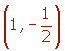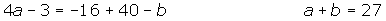Learn from home

The teachers## Exercise 1

Is Rolle's theorem applicable to the function f(x) = |x − 1| on the interval [0, 2]?

## Exercise 2

Determine if the function f(x) = x − x³ satisfies the conditions of Rolle's theorem on the interval [−1, 0] and [0, 1]. In the affirmative case, determine the values of c.

## Exercise 3

Does the function f(x) = 1 − x satisfy the conditions of Rolle's theorem on the interval [−1, 1]?

## Exercise 4

Prove that the equation 1 + 2x + 3x² + 4x³ = 0 has a unique solution.

## Exercise 5

How many roots does the equation x³ + 6x² + 15x − 25 = 0 have?

## Exercise 6

Prove that the equation 2x³ − 6x + 1 = 0 has only one real solution on the interval (0, 1).

## Exercise 7

Can the mean value theorem be applied to f(x) = 4x² − 5x + 1 on [0, 2]?

## Exercise 8

Can the mean value theorem be applied to f(x) = 1/ x² on [0, 2]?

## Exercise 9

In the segment of the parabola between the points A = (1, 1) and B = (3, 0), find a point whose tangent is parallel to the chord.

## Exercise 10

Calculate a point on the interval [1, 3] in which the tangent to the curve y = x³ − x² + 2 is parallel to the line determined by the points A = (1, 2) and B = (3, 20). What theorem guarantees the existence of this point?

## Exercise 11

Determine a and b for the function:If it satisfies the hypothesis of mean value theorem on the interval [2, 6].

## Solution of exercise 1

Is Rolle's theorem applicable to the function f(x) = |x - 1| on the interval [0, 2]?The function is continuous on [0, 2].

Rolle's Theorem is not applicable to the function because it is not differentiable at x = 1.## Solution of exercise 2

Determine if the function f(x) = x − x³ satisfies the conditions of Rolle's theorem on the interval [−1, 0] and [0, 1]. In the affirmative case, determine the values of c.

f(x) is a continuous function on the interval [−1, 0] and [0, 1], and differentiable on the open intervals (−1, 0) and (0, 1) because it is a polynomial function.

Also, it is determined that:

f(−1) = f(0) = f(1) = 0

Therefore, Rolle's Theorem is applicable.## Solution of exercise 3

Does the function f(x) = 1 − x satisfy the conditions of Rolle's Theorem on the interval [−1, 1]?

The function is continuous on the interval [−1, 1] and differentiable on (−1, 1) because it is a polynomial function.

Rolle's Theorem is not satisfied because f(−1) ≠ f(1).

## Solution of exercise 4

Prove that the equation 1 + 2x + 3x² + 4x³ = 0 has a unique solution.

It can be proven by the reductio ad absurdum arguement.

If the function had two different roots x1 and x2, being x1< x2 , there would be:

f(x1) = f(x2) = 0

And since the function is continuous and differentiable (as it is a polynomial function), Rolle's theorem can be applied, which states that c(x1, x2) exists such that f' (c) = 0.

f' (x) = 2 + 6x + 12x² f' (x) = 2 (1+ 3x + 6x²).

But f'(x) ≠ 0 and does not admit real solutions because the discriminant is negative:

Δ = 9 − 24 < 0.

Since the derivative is not annulled at any value, it contradicts Rolle´s theorem and it is determined that there are two false roots.

## Solution of exercise 5

How many roots does the equation x³ + 6x² + 15x − 25 = 0 have?

The function f(x) = x³ + 6x² + 15x − 25 is continuous and differentiable on·

f(0) = −25

f(2) = 37

So the equation has at least one solution in the interval (0, 2).

Rolle's theorem.

f' (x) = 3x² + 12x +15

Since the derivative is not annulled as its discriminant is negative, the function is strictly increasing and has a single root.

## Solution of exercise 6

Prove that the equation 2x³ − 6x + 1 = 0 has only one real solution on the interval (0, 1).

The function f(x) = 2x³ − 6x + 1 is continuous and differentiable on·

f(0) = 1

f(1) = −3

So, the equation has at least one solution in the interval (0, 1).

Rolle's theorem.

f' (x) = 6x² - 6 6x² - 6 = 0 6(x − 1) (x + 1) = 0

The derivative is annulled at x = 1 and x = −1, therefore there cannot be two roots in the interval (0, 1).

## Solution of exercise 7

Can the mean value theorem be applied to f(x) = 4x² − 5x + 1 on [0, 2]?

f(x) is continuous on [0, 2] and differentiable on (0, 2) therefore it is possible to apply the intermediate value theorem:## Solution of exercise 8

Can the mean value theorem be applied to f(x) = 1/ x² on [0, 2]?

The function is not continuous on [−1, 2] since is not defined at x = 0.

## Solution of exercise 9

In the segment of the parabola between the points A = (1, 1) and B = (3, 0), find a point whose tangent is parallel to the chord.

The points A = (1, 1) and B = (3, 0) belong to the parabola of equation y = x² + bx + c.Since the function is a polynomial, the mean value theorem can be applied on the interval [1, 3].## Solution of exercise 10

Calculate a point on the interval [1, 3] in which the tangent to the curve y = x³ − x² + 2 is parallel to the line determined by the points A = (1, 2) and B = (3, 20). What theorem guarantees the existence of this point?

Find the equation of the straight line that passes through the two points.Since y = x³ − x² + 2 is continuous on [1, 3] and differentiable on (1, 3), the intermediate value theorem can be applied:## Solution of exercise 11

Determine a and b for the function:If it satisfies the hypothesis of the mean value theorem on the interval [2, 6].

First, it must fulfill that the function is continuous on [2, 6].Second, it must be determined that the function is differentiable on (2, 6).Did you like the article?(1 votes, average: 5.00 out of 5)Loading...

Emma

I am passionate about travelling and currently live and work in Paris. I like to spend my time reading, gardening, running, learning languages and exploring new places.

Did you like
this resource?

Bravo!